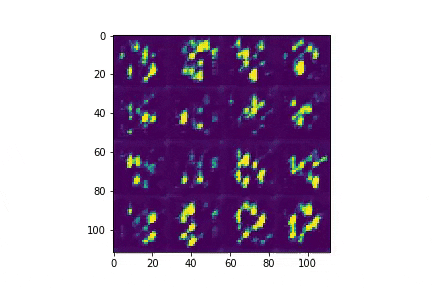GANs 2/nContinuing the last post, I'm moving on to DCGANs. The setup here (detailed below) is pretty standard, so I think the most intersting part is what didn't work; I'll jump straight to that.

Things I learned: Z's Shape Matters

If the first conv layer of your generator is nn.ConvTranspose2d, what's going to work better for your entropy source z? Is it

1. z.shape == (10,10,1) with in_dim == 1, or
2. z.shape == (100, 1) with in_dim == 100?

The answer is . This feels obvious in retrospect, but I mistakenly started out with . Here's the kind of samples you get after 10 epochs:More specifically, these are the kind of samples you get if your generator looks like this:

class G(nn.Module):

def __init__(self):
super().__init__()
self.conv_layers = nn.Sequential(
nn.ConvTranspose2d(  1, 64, 4, 1, 4),          # This doesn't work
nn.BatchNorm2d(64),
nn.ReLU(True),
nn.ConvTranspose2d( 64, 16, 4, 2, 0),  # 12
nn.BatchNorm2d(16),
nn.ReLU(True),
nn.ConvTranspose2d( 16,  4, 4, 2, 0),  # 26
nn.BatchNorm2d(4),
nn.ReLU(True),
nn.ConvTranspose2d(  4,  1, 3, 1, 0),  # 28
nn.Tanh()
)

def forward(self, z):
return self.conv_layers(z)

instead of like this:

class G(nn.Module):

def __init__(self):
super().__init__()
self.conv_layers = nn.Sequential(
nn.ConvTranspose2d(100, 64, 5, 2, 0),        # This works well
nn.BatchNorm2d(64),
nn.ReLU(True),
nn.ConvTranspose2d( 64, 16, 4, 2, 0),  # 12
nn.BatchNorm2d(16),
nn.ReLU(True),
nn.ConvTranspose2d( 16,  4, 4, 2, 0),  # 26
nn.BatchNorm2d(4),
nn.ReLU(True),
nn.ConvTranspose2d(  4,  1, 3, 1, 0),  # 28
nn.Tanh()
)

def forward(self, z):
return self.conv_layers(z)

The first layer is the only thing that's different. Anyway, the samples are not that great, right? I have a couple guesses about this. First, there's the number of weights: the "bad" setup has 1x64x4x4 and the "good" setup has 100x64x5x5. So the better setup has more capacity to learn.

Second, there's the weight sharing. My understanding is that for each of the "bad" setup's 64 channels, we're learning a single 4x4 window that expands a 10x10 input into a 13x13 output, and then cuts off the edges (the opposite of padding) to get a 5x5 center. For each of the "good" setup's 64 channels, we're learning a 5x5x100 window that expands our 1x100 input into a 5x5 output. This feels like it should be able to use our source of entropy more flexibly / with less premature projection.

Training Setup

Here's the setup that I used in the end. The network for the generator looks like this:

class G(nn.Module):

def __init__(self):
super().__init__()
self.conv_layers = nn.Sequential(
nn.ConvTranspose2d(100, 64, 5, 2, 0),
nn.BatchNorm2d(64),
nn.ReLU(True),
nn.ConvTranspose2d( 64, 16, 4, 2, 0),  # 12
nn.BatchNorm2d(16),
nn.ReLU(True),
nn.ConvTranspose2d( 16,  4, 4, 2, 0),  # 26
nn.BatchNorm2d(4),
nn.ReLU(True),
nn.ConvTranspose2d(  4,  1, 3, 1, 0),  # 28
nn.Tanh()
)

def forward(self, z):
return self.conv_layers(z)

And the network for D looks like this:

def __init__(self):
super().__init__()
self.conv_layers = nn.Sequential(
nn.Conv2d(1, 4, 3, 1, 0),              # (28-3)/1+1 = 26
nn.LeakyReLU(0.2, inplace=True),
nn.BatchNorm2d(4),
nn.Conv2d(4, 16, 4, 2, 0),              # (26-4)/2+1 = 12
nn.LeakyReLU(0.2, inplace=True),
nn.BatchNorm2d(16),
nn.Conv2d(16, 64, 4, 2, 0),              # (12-4)/2+1 = 5
nn.LeakyReLU(0.2, inplace=True),
nn.BatchNorm2d(64),
nn.Conv2d(64, 256, 5, 2, 0),             # (5-5)/2+1 = 1
)
self.linear_layers = nn.Sequential(
nn.Linear(16*16, 16),
nn.Linear(16, 1),
nn.Sigmoid()
)

def forward(self, x):
bs = x.shape
y1 = self.conv_layers(x)
y2 = self.linear_layers(y1.view(bs, -1))
return y2

The training loop is standard, and identical to the last post.

I used the Adam optimizer for both G and D (default betas, learning rate of 3e-4), and optimized against BCELoss. I trained a Colab notebook with a GPU backend (I believe it's a T4 as of 2019-05-19). Code also available here.

Results

The resulting samples look decent after 50 epochs.There are some obvious remaining imperfections. For example, the figure in row 3, column 3 doesn't really converge to something that looks like a digit. To my eye, the strokes look more natural and less static-filled than the ones from the vanilla GAN

Here are the losses by epoch:And here are the mean discriminator scores (0 for fake, 1 for real):